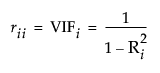For the latest version of JMP Help, visit JMP.com/help.

Publication date: 11/29/2021

## Inverse Correlation Matrix

The inverse correlation matrix provides useful multivariate information. The diagonal elements of the inverse correlation matrix, sometimes called the variance inflation factors (VIF), are a function of how closely the variable is a linear function of the other variables. Specifically, if the correlation matrix is denoted R and the inverse correlation matrix is denoted R-1, the diagonal element is denoted rii and is computed as follows:where Ri2 is the coefficient of variation from the model regressing the ith explanatory variable on the other explanatory variables. Thus, a large rii indicates that the ith variable is highly correlated with any number of the other variables.

Want more information? Have questions? Get answers in the JMP User Community (community.jmp.com).